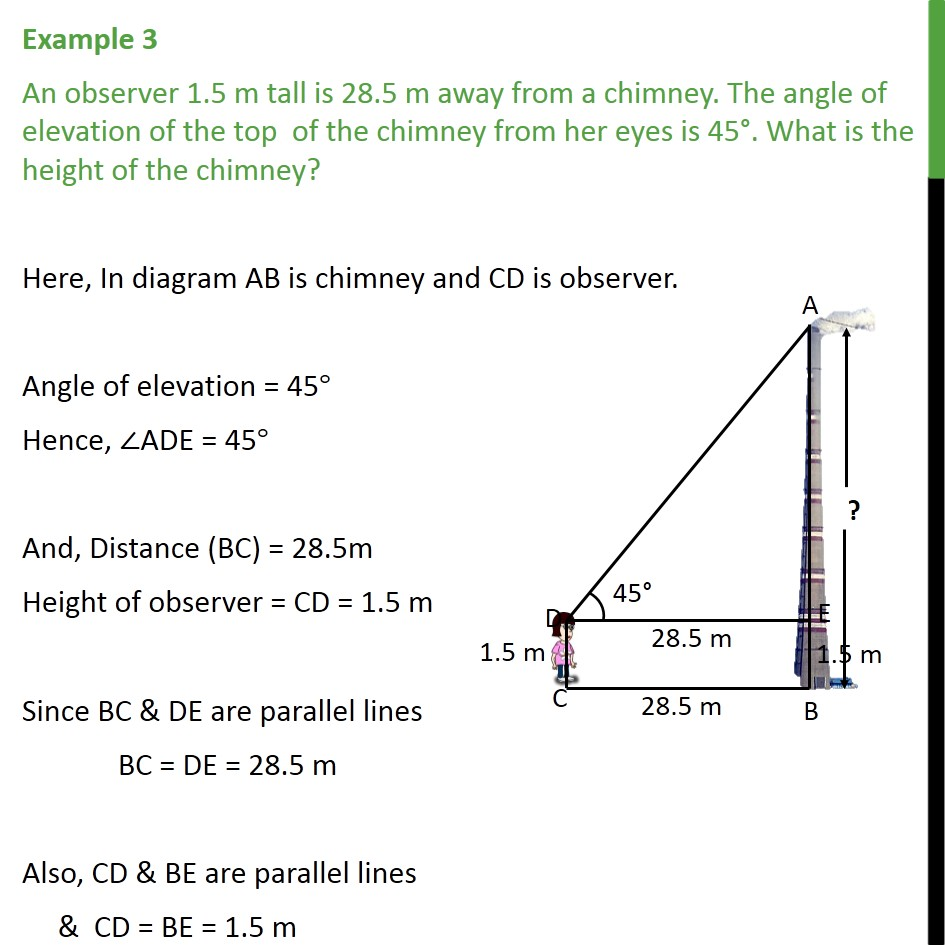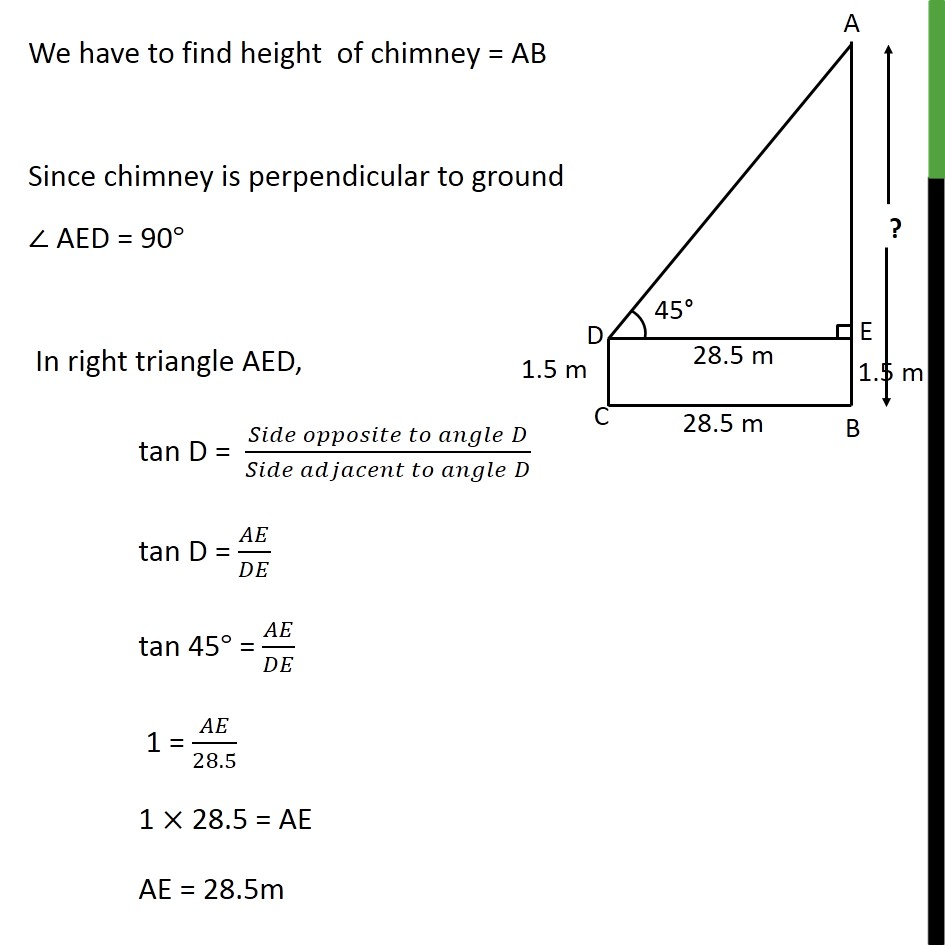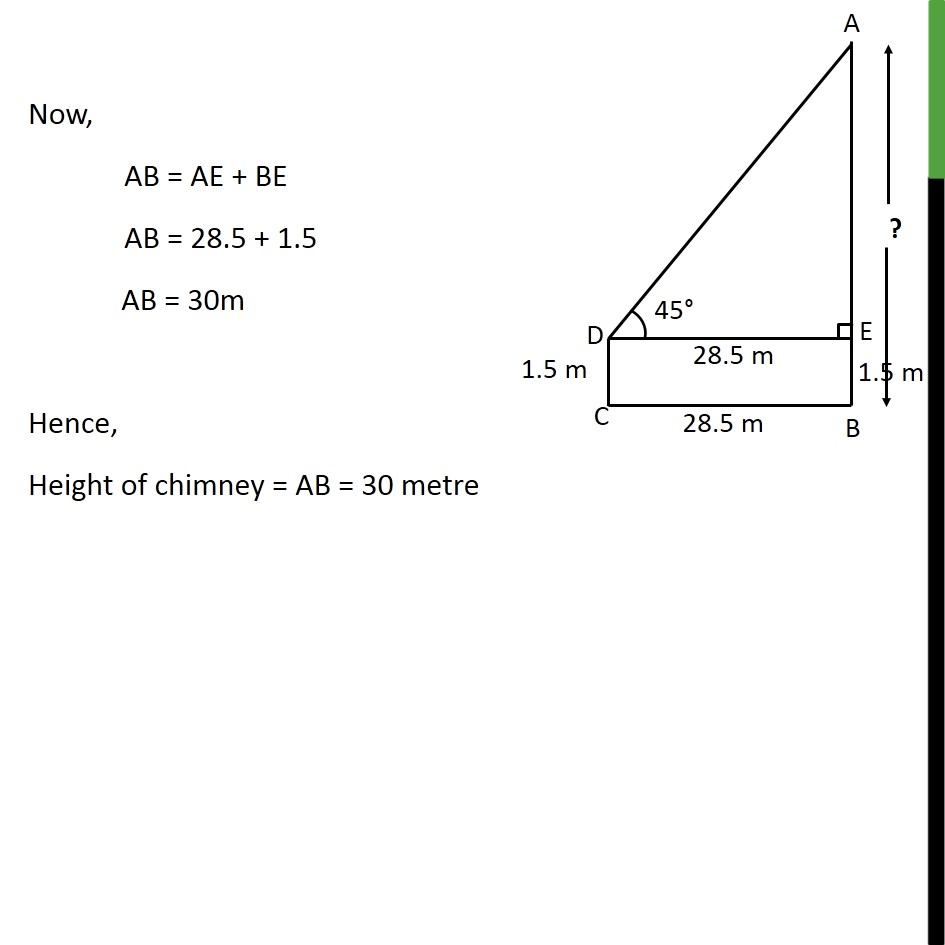1. Chapter 9 Class 10 Some Applications of Trigonometry (Term 2)
2. Concept wise
3. Questions easy to difficult

Transcript

Example 3 An observer 1.5 m tall is 28.5 m away from a chimney. The angle of elevation of the top of the chimney from her eyes is 45°. What is the height of the chimney? Here, In diagram AB is chimney and CD is observer. Angle of elevation = 45° Hence, ∠ADE = 45° And, Distance (BC) = 28.5m Height of observer = CD = 1.5 m Since BC & DE are parallel lines BC = DE = 28.5 m Also, CD & BE are parallel lines & CD = BE = 1.5 m We have to find height of chimney = AB Since chimney is perpendicular to ground ∠ AED = 90° In right triangle AED, tan D = (𝑆𝑖𝑑𝑒 𝑜𝑝𝑝𝑜𝑠𝑖𝑡𝑒 𝑡𝑜 𝑎𝑛𝑔𝑙𝑒" " 𝐷)/(𝑆𝑖𝑑𝑒 𝑎𝑑𝑗𝑎𝑐𝑒𝑛𝑡 𝑡𝑜 𝑎𝑛𝑔𝑙𝑒" " 𝐷) tan D = 𝐴𝐸/𝐷𝐸 tan 45° = 𝐴𝐸/𝐷𝐸 1 = 𝐴𝐸/28.5 1 × 28.5 = AE AE = 28.5m Now, AB = AE + BE AB = 28.5 + 1.5 AB = 30m Hence, Height of chimney = AB = 30 metre